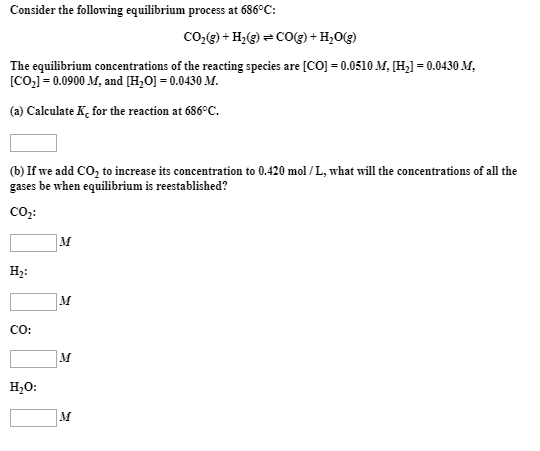# Consider the following equilibrium process at 686 °C: CO2(g) + H2(g) ⇌ Co(g) + H2O(g) The equilibrium concentrations of the reacting species are [CO] = 0.0510 M, [H2] = 0.0430 M, [CO2] = 0.0900 M, and [H2)] = 0.0430 M. (a) Calculate Kc for the reaction at 686 °C. (b) If we add CO2 to increase its concentration to 0.420 mol/L, what will the concentrations of all the gases be when equilibrium is reestablished?